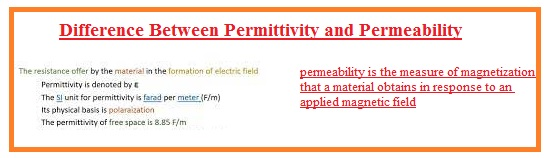Hello, friends, I hope you all are doing great. In today’s tutorial, we will discuss the Difference Between Permittivity & Permeability. The basic difference between permittivity and permeability is that calculates the hindrance offered by the substance in the creation of an electric field. While permeability explains the capability of substance that permits to flow of magnetic field lines in the material. On polarisation of substance, permittivity depends and permeability depends on the magnetism.

In today’s post, we will have a detailed look at both permittivity and permeability with the details and compare them to find their differences. So let’s get started with Difference Between Permittivity & Permeability.

#### Difference Between Permittivity & Permeability

Permittivity

• It is the behavior that shows the polarizing feature of any dielectric material
• The material that has a high value of permittivity is more polar and give a good response to the field given
• Permittivity is a factor that helps to measure the value of capacitance of any capacity
• It is denoted with the ε.
• Its mathematical expression can be formed by the ratio between displacement field strength and electric field strength.
• The system’s international unit of permittivity is Faraday/meter. and C/V.m
• The phenomena of permittivity is used in a capacitor.
• Its physical dependency is on Polarization.
• The value of free space permittivity is  8.85 F/m.
• the permittivity of material with respect to space is called relative permittivity denoted as εr and also called the dielectric constant of that material

Permeability

• The ability of any substance that explains the movement of magnetic field lines in that material is called permeability.
• It is denoted with the μ.
• Its mathematical expression can give by taking the ratio between magnetic field density and magnetic field strength.
• Its system international unit is Henry/meter. also measured in newton per amper square
• It used in Transformer core to study the flux behavior
• The first time it was introduced by the Oliver Heaviside
• Its inverse is called reluctivity
• Its constant called magnetic contact used for the permeability of free space.
• The related term of permeability ios magnetic susceptibility
• his phenomenon is based on Magnetization.
• The value of permeability of free space is 1.26 H/m.
• The field is generated by the material due to diamagnetism when the outer field is linked to it.
• Such material has a permeability value of less than one
• Material having permeability value larger than one called diamagnetThat is detailed post about the difference between permittivity and permeability if you have any query ask in comments. Thanks for reading. Have a good day.